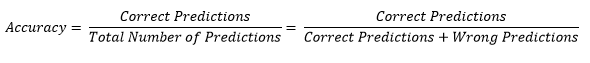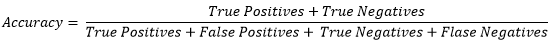Classification

Logistic Regression

Machine Learning

December 31, 2020

# What is Accuracy?

Accuracy of a machine learning model is the ratio of correct predictions to all the predictions made.The formula for accuracy using the metrics of Confusion Matrix would be.by : Monis Khan

Quick Summary:

Accuracy of a machine learning model is the ratio of correct predictions to all the predictions made. The formula for accuracy using the metrics of Confusion Matrix would be.